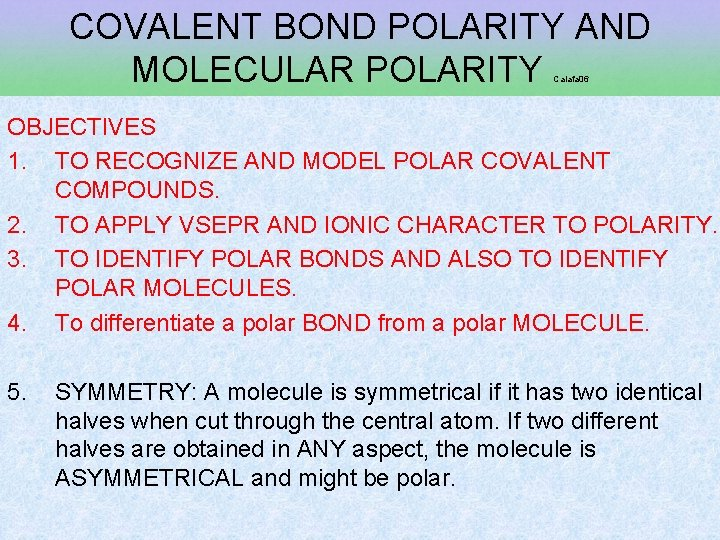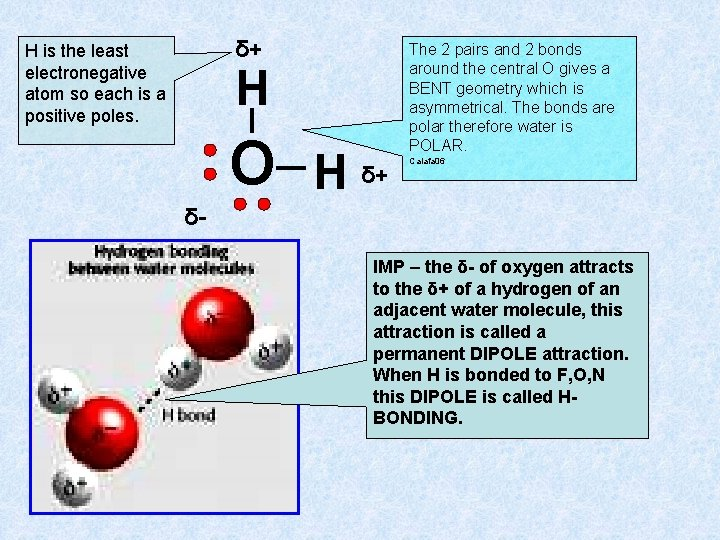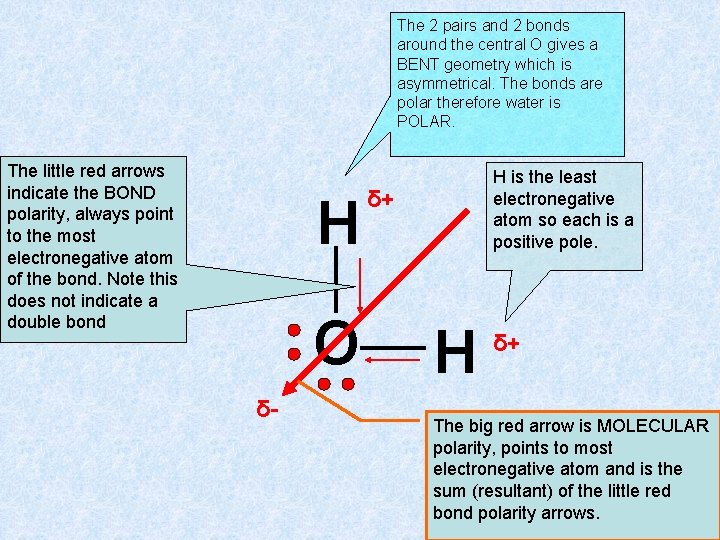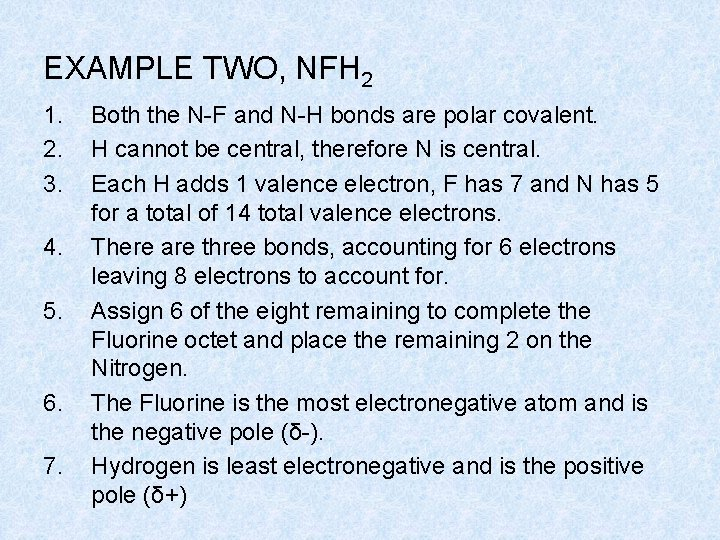# COVALENT BOND POLARITY AND MOLECULAR POLARITY Caiafa 06

• Slides: 7
Download presentationCOVALENT BOND POLARITY AND MOLECULAR POLARITY Caiafa 06 OBJECTIVES 1. TO RECOGNIZE AND MODEL POLAR COVALENT COMPOUNDS. 2. TO APPLY VSEPR AND IONIC CHARACTER TO POLARITY. 3. TO IDENTIFY POLAR BONDS AND ALSO TO IDENTIFY POLAR MOLECULES. 4. To differentiate a polar BOND from a polar MOLECULE. 5. SYMMETRY: A molecule is symmetrical if it has two identical halves when cut through the central atom. If two different halves are obtained in ANY aspect, the molecule is ASYMMETRICAL and might be polar.1. 2. 3. 4. A bond is considered POLAR if the electrons are not shared equally as indicated by an ionic character above about 0. 4 and below 1. 7. In a bond between two atoms, the more electronegative atom is the NEGATIVE POLE and pulls the electrons closer to itself but does not ever gain them – no ions are formed. The less electronegative atom has the electrons pulled further away from it without the loss of electrons or the formation of positive ions, the less electronegative atom is the POSITIVE POLE of the bond. A molecule can only be termed polar if: 1. 2. It has polar bonds The bonds are ASYMMETRICAL around the central atom(VSEPR)SKILL- PREDICTING POLARITY 1. STEP ONE: calculate ionic character or EACH type of bond in the molecule. 2. STEP TWO: render a VSEPR Lewis dot structure, assess symmetry. 3. STEP THREE: assign the least electronegative atom as the positive pole δ+. 4. Assign the most electronegative atom as the negative pole δ-. 5. Using the VSEPR charts, determine the geometry and symmetry. 6. IF the molecule is ASYMMETRICAL and the bonds are POLAR, the molecule is polar. EXAMPLE ONE, WATER H 2 O STEP ONE: the ionic character of water is 3. 5 – 2. 2 = 1. 3 which is a means the O to H bond is POLAR COVALENT. STEP TWO: • The oxygen has 6 valence electrons and each H has one each for a total of 8 valence electrons. • Two bonds are needed to connect the H atoms to the O, there are 4 bonded electrons which leaves 4 remaining. Remember H cannot be central and never gets dots in any VSEPR structure. • The remaining four electrons go on O as H is not an octet element. This gives two bonds and 2 pairs on the central O for a BENT geometry, which is asymmetrical.δ+ H is the least electronegative atom so each is a positive poles. H O H δ+ The 2 pairs and 2 bonds around the central O gives a BENT geometry which is asymmetrical. The bonds are polar therefore water is POLAR. Caiafa 06 δIMP – the δ- of oxygen attracts to the δ+ of a hydrogen of an adjacent water molecule, this attraction is called a permanent DIPOLE attraction. When H is bonded to F, O, N this DIPOLE is called HBONDING.The 2 pairs and 2 bonds around the central O gives a BENT geometry which is asymmetrical. The bonds are polar therefore water is POLAR. The little red arrows indicate the BOND polarity, always point to the most electronegative atom of the bond. Note this does not indicate a double bond H O δ- H is the least electronegative atom so each is a positive pole. δ+ H δ+ The big red arrow is MOLECULAR polarity, points to most electronegative atom and is the sum (resultant) of the little red bond polarity arrows.EXAMPLE TWO, NFH 2 1. 2. 3. 4. 5. 6. 7. Both the N-F and N-H bonds are polar covalent. H cannot be central, therefore N is central. Each H adds 1 valence electron, F has 7 and N has 5 for a total of 14 total valence electrons. There are three bonds, accounting for 6 electrons leaving 8 electrons to account for. Assign 6 of the eight remaining to complete the Fluorine octet and place the remaining 2 on the Nitrogen. The Fluorine is the most electronegative atom and is the negative pole (δ-). Hydrogen is least electronegative and is the positive pole (δ+)8 The molecule is 3 bonds and one pair on the central atom, It is therefore pyramidal and asymmetrical. 9 This is an asymmetrical molecule with polar bonds and is therefore polar. δ- F N H δ+